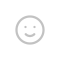## 常规算法

print("素数的概念是只可以被1和它本身整除的数字。\n欢迎来到这里，我们将在这里计算你所输入的数字是否为素数。")
while True:
number = input("输入你的数字吧：")
number = int(number)
if number == 2:
print("是素数")
elif number == 3:
print("是素数")
elif number == 5:
print("是素数")
elif number == 7:
print("是素数")
elif number == 11:
print("是素数")
elif number == 17:
print("是素数")
elif number == 13:
print("是素数")
elif number == 19:
print("是素数")
else:
if number % 2 == 0:
print("\t此数可以被2整除，因此不是素数。")
else:
if number % 3 == 0:
print("\t此数可以被3整除，因此不是素数。")
else:
if number % 5 == 0:
print("\t此数可以被5整除，因此不是素数。")
else:
if number % 7 == 0:
print("\t此数可以被7整除，因此不是素数。")
else:
if number % 11 == 0:
print("\t此数可以被11整除，因此不是素数。")
else:
if number % 13 == 0:
print("\t此数可以被13整除，因此不是素数。")
else:
if number % 17 == 0:
print("\t此数可以被17整除，因此不是素数。")
else:
if number % 19 == 0:
print("\t此数可以被19整除，因此不是素数。")
else:
print("是素数")

## 高阶算法

print("提示：最终结果的显示时间取决与CPU的算力和你输入的数大小\n建议输入一千万以下的数字，数字太大无法在短时间内得出结果")
x = int(input('输入一个数：'))
z = 0
for i in range(1,x+1):
if x % i == 0:
z = z+1
if z > 2:
print("不是素数")
else:
print("是素数")

## 代码分析

#### 使用 int() 进行数字转换

int() 函数在高阶算法中有使用，我们使用 int()input() 嵌套，不必再多写一行代码来转换，其作用和 float() 函数类似，但是它不会使得数字转化为浮点数，而是一个实实在在的数。

a = "2"
b = int(a)
print(b)
c = b+1
print(b)
print(c)

2
2
3

#### 使用 range() 来生成数

for a in range(1,10):
print(a)

1
2
3
4
5
6
7
8
9

## 评论

1.Windows Chrome
1年前
2022-7-25 15:54:15

Hello!建议博主之后有空可以修改下这篇blog😀。

•Magneto
博主
Windows Edge
1年前
2022-7-25 19:45:40

很好奇，这该怎么改

## 发送评论编辑评论Emoji